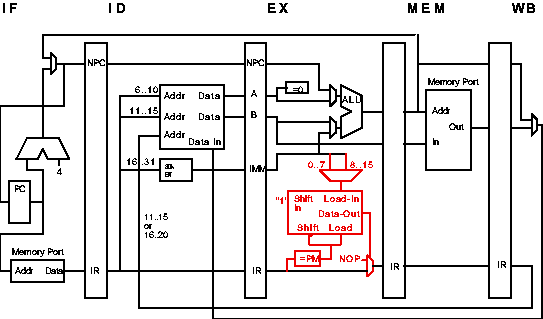# EE 4720 Computer Architecture - HW 3 Solution

### Problem 1

Conventional implementation. If r10=0, skip every other instruction:
``` beqz r10,A
A:
beqz r10,B
B:
beqz r10,C
C:
beqz r10,D
D:
```
(Note that a good programmer would implement the code fragment above differently.)

Notice that all branches use the some register. That makes it easy to replace all branches with a single PM:

``` pm R10, 0xFF, 0xAA
```

### Problem 2

Consider execution of the first four instructions of the conventional code fragment when r10=0. The add instruction, which started execution under the predict-not-taken assumption, is abandoned. The addi instruction starts three cycles after the IF for the add. The process repeats with the second beqz instruction.

```
0  1   2   3   4   5   6   7
beqz  IF  ID  EX  MEM WB
A:
addi		       IF  ID  EX  MEM WB
beqz			   IF  ID  EX  MEM WB

```
The time between the beqz instructions is five cycles, the number of instructions executed is 2. This sequence repeats four times in the entire code fragment, and the sequence takes another four cycles to complete (for the pipeline to drain. So:

IC = 2 × 4 = 8.
t / cycles = 2 × 5 + 4 = 24.
CPI = 24 / 8 = 3

With the PM instruction there are no stalls, so the total execution time is 9 + 4 = 13 cycles. When r10=0, four instructions are nulled, so IC = 5. Then

CPI = 13 / 5 = 2.6

The execution time is almost twice as good (which is what counts), and the CPI is slightly better.

### Problem 3

The solution below is incomplete: it will not work properly with control-transfer instructions, nor will it work if the pipeline stalls. (The complete solution might give away a future homework assignment.)

As shown in red below, add an eight-bit shift register to the EX stage. When a PM instruction is in EX, the appropriate 8-bit execution mask (from the immediate portion of the ID/EX latch) is loaded into the shift register.

At every cycle, the least significant bit of the shift register is checked. If it's zero, the instruction in the EX stage is nulled by replacing it with a NOP. (This works because instructions do not change "state" until the MEM and WB stages.) At the end of the cycle the shift register is shifted right (removing the least significant bit) with a 1 shifted into the left side.### Problem 4

If loads were allowed, then operation of the shift register would be complicated by stall cycles (but this would not be too great a problem). Allowing branches would make programming tricky since instructions at the branch target would be affected by the execution mask. The PM description did not describe what would happen if a PM were encountered within 8 instructions of another PM. One possibility is that the mask in the second PM could overwrite the first, assuming the second PM was not nullified itself.

Things would be simplest if only arithmetic (including logical and compare) instructions were allowed after a PM. So a reasonable restriction is that the PM works as described above until a non-arithmetic instruction is encountered, in which case the remainder of the mask is set to ones (i.e., the PM instruction is cancelled) or reloaded with a new mask if the non-arithmetic instruction is another PM.David M. Koppelman - koppel@ee.lsu.edu Modified 4 Mar 1997 19:37 (1:37 UTC)